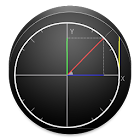Unit Circle (Trigonometric)

All Android applications categories

All Android games categories# Unit Circle (Trigonometric)

177 8.4

8.4 Users
rating

## Screenshots

Description

The unit circle or trigonometric circle is a circle whose center is located at the origin of the Cartesian plane and its radius is 1 (one). It is used in the study of trigonometric functions such as sine, cosine and tangent.

- Understand how the projections of the arcs sine, cosine and tangent work in the unit circle (or trigonometric circle);

- Why the values ​​of sine and cosine are limited to -1 and +1;

- Why the tangent of 90 ° and 270 ° are not defined

- When and where the values ​​of the trigonometric ratios (sine, cosine, tangent) are positive, negative, zero or null;

-------------------------------------------------------------------------
If you have any suggestion fell free to contact me.

or

If you get any error or problem running the app. Send me the report and some message. So I get more information about your android version, screen size, ... so I can fix it. Thanks.

from 177 reviews

"Great"

8.4